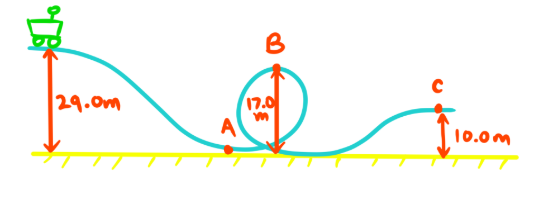# Conservation of energy

### Conservation of energy

#### Lessons

In this lesson, we will learn:
• Conservation of mechanical energy
• When to use conservation of energy to solve problems

Notes:

• Energy is a conserved quantity: it can change forms and be transferred from one place to another, but it cannot be created or destroyed. For a process where energy changes forms or gets transferred, we can say that total energy before the process is equal to total energy after, or $\sum E_i = \sum E_f$
• Mechanical energy is energy that an object has due to its motion and position. It is the sum of the object's kinetic energy ($E_k$) and potential energy ($E_p$).
• In real world processes, the mechanical energy an object possesses can be "lost" to other non-mechanical forms of energy. (The energy is lost in the sense that it is no longer in the object, but it is not destroyed since it gets transferred to the object's environment.)
• For example, a box that is sliding across the floor has a certain amount kinetic energy, but eventually will come to a stop due to friction. The friction between the box and the floor converts the kinetic energy of the box to heat energy. When the box comes to a stop, all of the kinetic energy it originally had has been converted to heat: the equation $\sum E_i = \sum E_f$ could be expressed as $E_{k\;initial} = E_{heat final}$ for this process
• Energy is always conserved, but a special case when solving problems is when an object doesn't lose or gain any mechanical energy to/from its environment during a process. In this case, rather than saying total energy of the object and its environment is conserved (which is always true), we can say the total energy of that particular object is conserved (which is only true sometimes). This means that the total mechanical energy it has before the process is the same as after, though the balance of kinetic and potential energy it has may change. The equation $\sum E_i = \sum E_f$ in these cases becomes $E_{ki} + E_{pi} = E_{kf} + E_{pf}$. A common type of process where this is true is objects that move under the influence of gravity without any other external forces that cause mechanical energy to be lost as heat (such as friction or air resistance).
• For example, imagine a book balanced right at the edge of a bookshelf with 10 J of stored gravitational potential energy, but no kinetic energy. If the book falls, the 10 J of potential energy would be converted to kinetic energy over the course of the fall. If air resistance is negligible, all the potential energy is converted to kinetic energy and the book would have 10 J of kinetic energy the moment before it hits the ground. Additionally, conservation of energy means that at any point during the fall the total energy of the book is 10 J: the sum of the book's kinetic energy (from its current speed) and potential energy (from its remaining height above the ground) always adds to 10 J.
• Once the book hits the ground, the energy of the book is no longer conserved, but total energy is conserved (as it always is). The kinetic energy of the book is dissipated as 10 J of heat during the collision with the ground.

Kinetic Energy

$E_k = \frac{1}{2}mv^2$

$E_k;$ kinetic energy, in joules (J)

$m:$ mass, in kilgrams (kg)

$v:$ velocity, in meters per second (m/s)

Gravitational Potential Energy

$E_p = mgh$

$E_p:$ gravitational potential energy, in joules (J)

$g:$ acceleration due to gravity, in meters per second squared (m/s2)

$h:$ height, in meters (m)

Conservation of Energy

$\sum E_i = \sum E_f$

$\sum E_i:$ sum of all initial energy, in joules (J)

$\sum E_f:$ sum of all final energy, in joules (J)

• 1.
$\bold{\sum E_i = \sum E_f:}$ Conservation of mechanical energy of a rollercoaster
You ride the pictured rollercoaster. Starting from rest, the cart receives a small push to send it down the slope. Assume the track is frictionless and there is no air resistance. What is your speed at each point A, B, and C?• 2.
$\bold{\sum E_i = \sum E_f:}$ Conservation of mechanical energy of a ball thrown straight up
A ball is thrown upwards with an initial velocity of 8.25 m/s [up] at a height of 1.00 m above the ground. What is the maximum height the ball will reach?